# Number Systems Decimal Binary Denary Octal Hexadecimal Click

• Slides: 43Number Systems Decimal Binary Denary Octal Hexadecimal Click the mouse or Press the space bar to ContinueExponents 20 = 1 l 21 = 2 l 22 = 2 x 2 =4 l 23 = 2 x 2 = 8 l x 5 + x 10 = x 15 l 1 / x 2 = x -2 l Click to Continue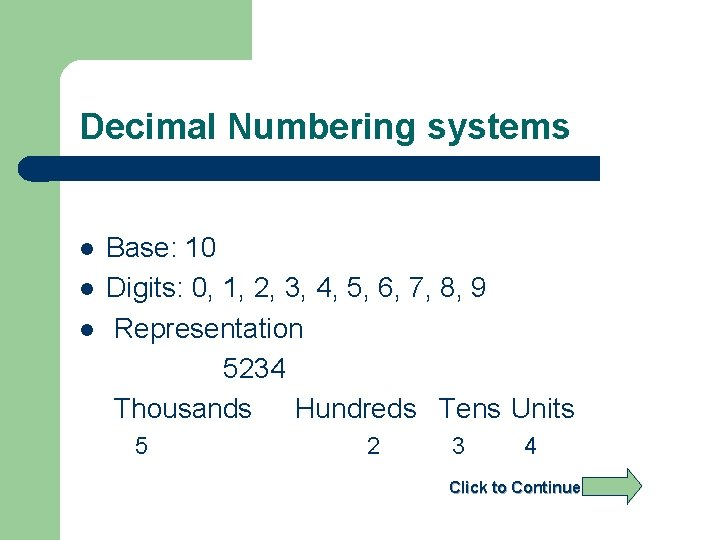Decimal Numbering systems l l l Base: 10 Digits: 0, 1, 2, 3, 4, 5, 6, 7, 8, 9 Representation 5234 Thousands Hundreds Tens Units 5 2 3 4 Click to Continue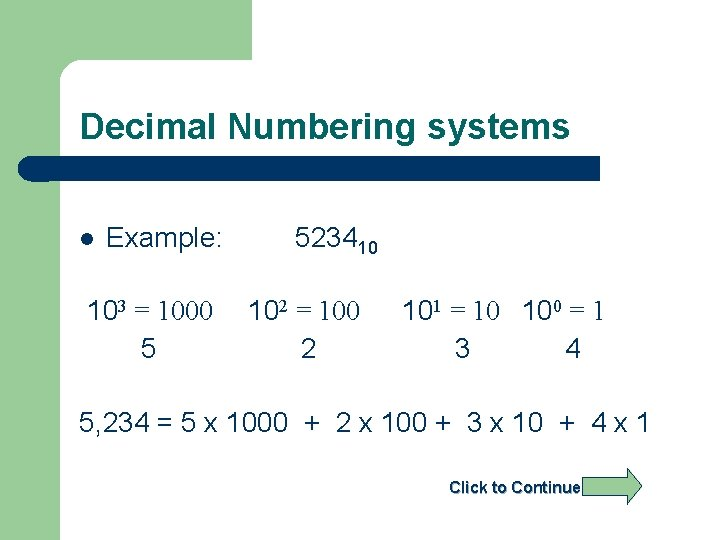Decimal Numbering systems l Example: 103 = 1000 5 523410 102 = 100 2 101 = 10 100 = 1 3 4 5, 234 = 5 x 1000 + 2 x 100 + 3 x 10 + 4 x 1 Click to Continue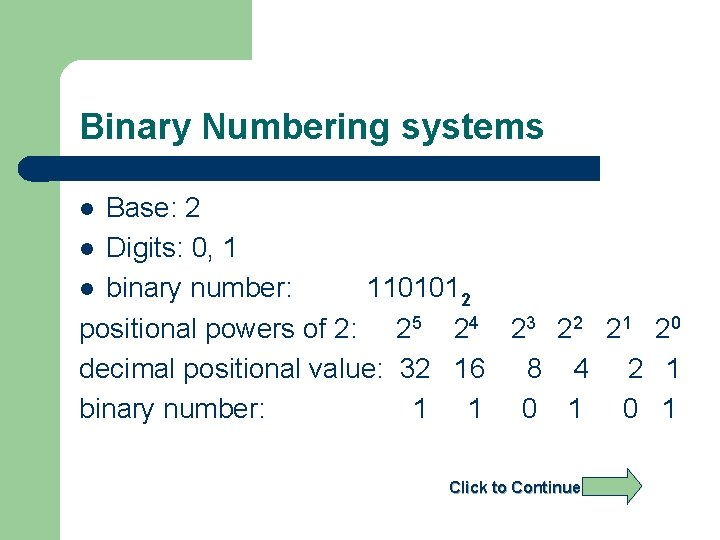Binary Numbering systems Base: 2 l Digits: 0, 1 l binary number: 1101012 positional powers of 2: 25 24 23 22 21 20 decimal positional value: 32 16 8 4 2 1 binary number: 1 1 0 1 l Click to Continue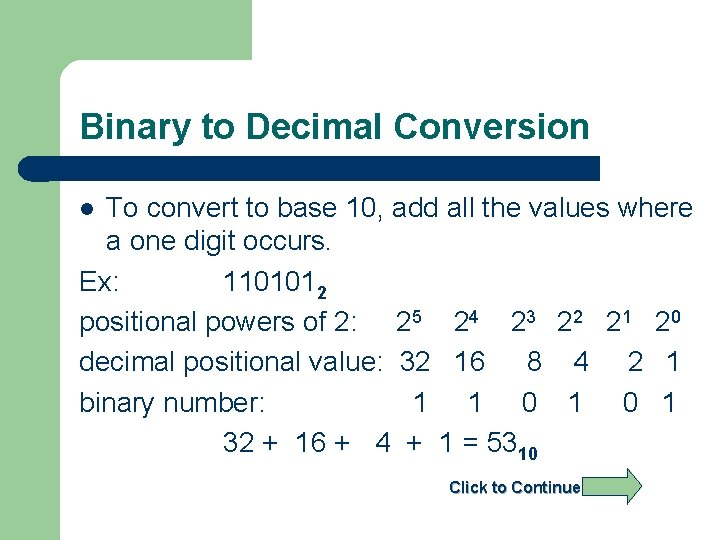Binary to Decimal Conversion To convert to base 10, add all the values where a one digit occurs. Ex: 1101012 positional powers of 2: 25 24 23 22 21 20 decimal positional value: 32 16 8 4 2 1 binary number: 1 1 0 1 32 + 16 + 4 + 1 = 5310 l Click to Continue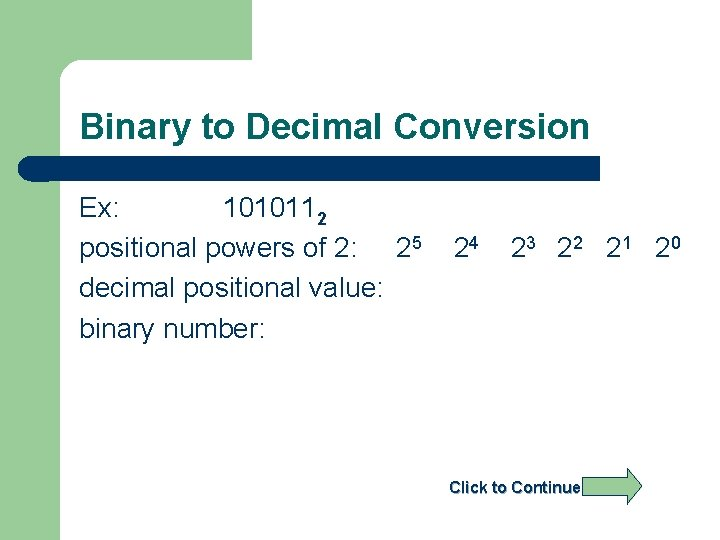Binary to Decimal Conversion Ex: 1010112 positional powers of 2: 25 decimal positional value: binary number: 24 23 2 2 2 1 2 0 Click to Continue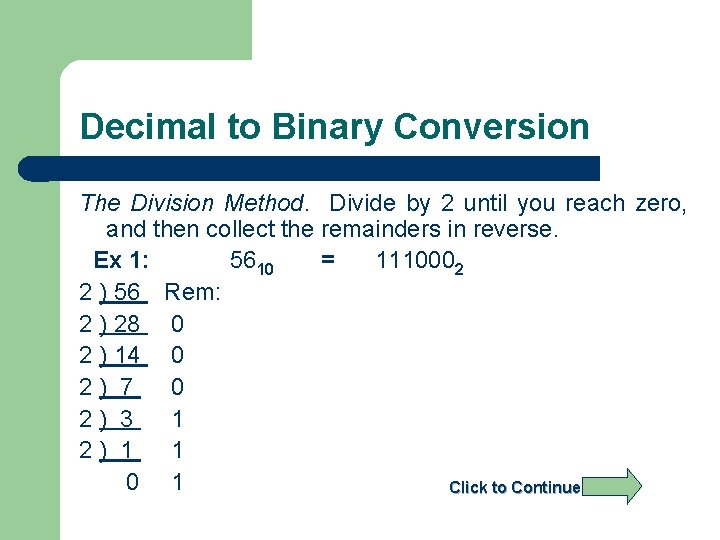Decimal to Binary Conversion The Division Method. Divide by 2 until you reach zero, and then collect the remainders in reverse. Ex 1: 5610 = 1110002 2 ) 56 Rem: 2 ) 28 0 2 ) 14 0 2) 7 0 2) 3 1 2) 1 1 0 1 Click to ContinueDecimal to Binary Conversion Ex 2: 3510 = 2) 2) 2) Rem: Answer: 3510 = 2 Click to Continue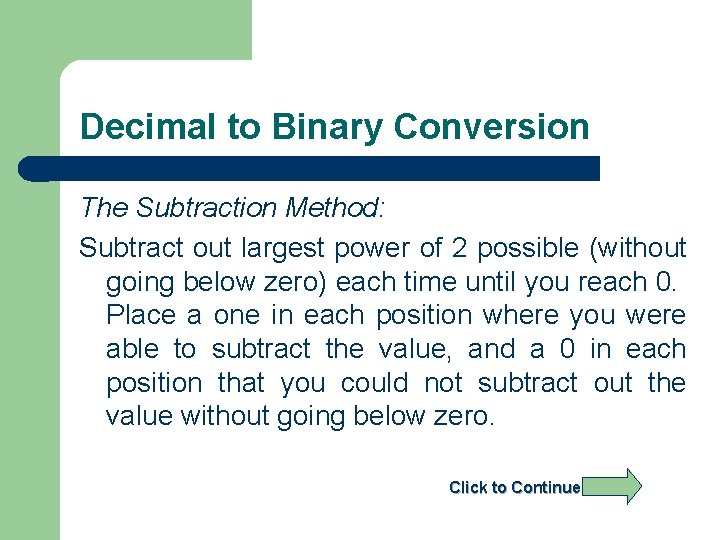Decimal to Binary Conversion The Subtraction Method: Subtract out largest power of 2 possible (without going below zero) each time until you reach 0. Place a one in each position where you were able to subtract the value, and a 0 in each position that you could not subtract out the value without going below zero. Click to ContinueDecimal to Binary Conversion Ex 1: 56 - 32 24 - 16 8 - 8 0 5610 26 | 2 5 24 23 22 21 20 64 | 32 16 8 4 2 1 | 1 1 1 0 0 0 Answer: 5610 = 1110002 Click to Continue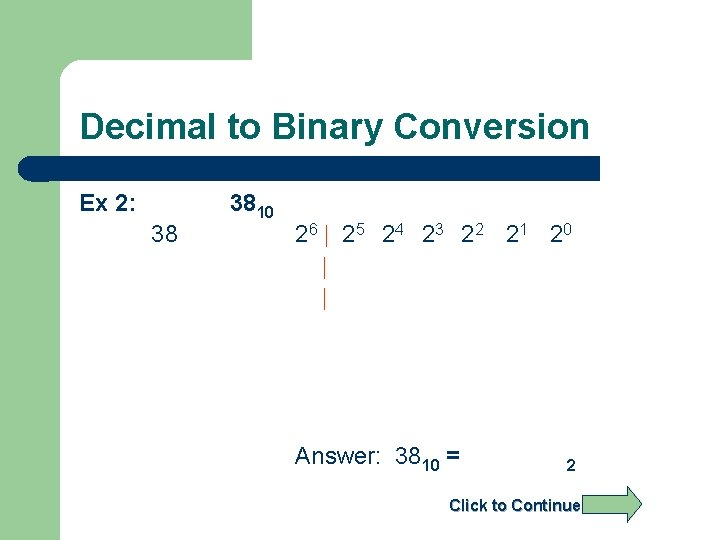Decimal to Binary Conversion Ex 2: 38 3810 26 | 2 5 24 23 22 21 20 | | Answer: 3810 = 2 Click to ContinueCharacter Representation ASCII Table Rightmost Four Bits 0000 0001 0010 0011 0100 0101 0110 0111 1000 1001 1010 1011 1100 1101 1110 1111 000 NUL SOH STX EOT ENQ ACK BEL BS HT LF VT FF CR SO SI 001 DLE DC 1 DC 2 DC 3 DC 4 NAK SYN ETB CAN EM SUB ESC FS GS RS US Leftmost Three Bits 010 011 100 Space 0 @ ! 1 A " 2 B # 3 C \$ 4 D % 5 E & 6 F ' 7 G ( 8 H ) 9 I * : J + ; K , < L = M. > N / ? O 101 P Q R S T U V W X Y Z [ ] ^ _ 110 ` a b c d e f g h I j k l m n o 111 p q r s t u v w x y z { | } ~ DEL Click to ContinueCharacter Representation Ex: Find the binary ASCII and decimal ASCII values for the ‘&’ character. Rightmost Four Bits 0000 0001 0010 0011 0100 0101 0110 0111 1000 1001 1010 1011 1100 1101 1110 1111 000 NUL SOH STX EOT ENQ ACK BEL BS HT LF VT FF CR SO SI 001 DLE DC 1 DC 2 DC 3 DC 4 NAK SYN ETB CAN EM SUB ESC FS GS RS US Leftmost Three Bits 010 011 100 Space 0 @ ! 1 A " 2 B # 3 C \$ 4 D % 5 E & 6 F ' 7 G ( 8 H ) 9 I * : J + ; K , < L = M. > N / ? O 101 P Q R S T U V W X Y Z [ ] ^ _ 110 ` a b c d e f g h I j k l m n o 111 p q r s t u v w x y z { | } ~ DEL Click to Continue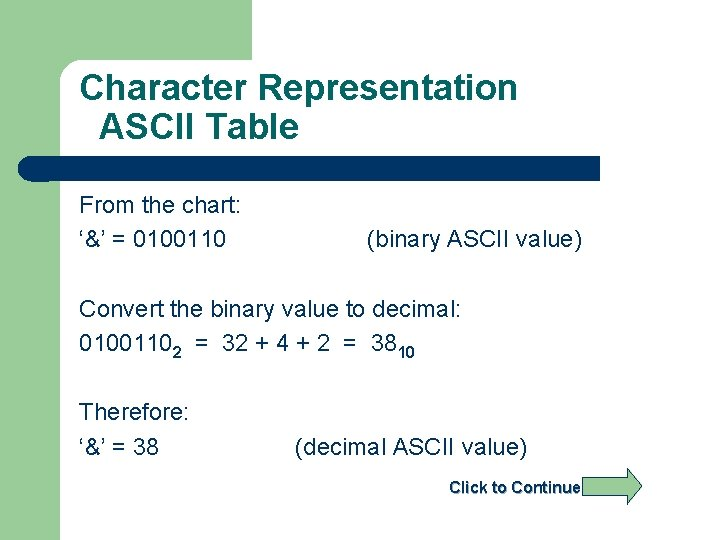Character Representation ASCII Table From the chart: ‘&’ = 0100110 (binary ASCII value) Convert the binary value to decimal: 01001102 = 32 + 4 + 2 = 3810 Therefore: ‘&’ = 38 (decimal ASCII value) Click to Continue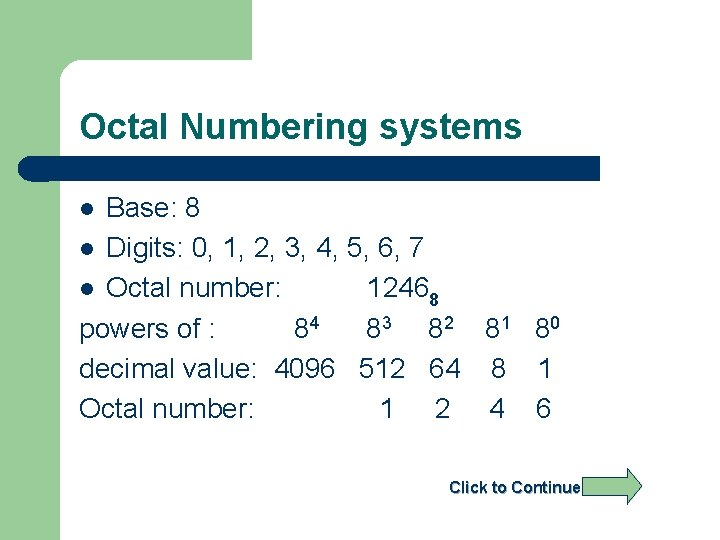Octal Numbering systems Base: 8 l Digits: 0, 1, 2, 3, 4, 5, 6, 7 l Octal number: 12468 powers of : 84 8 3 8 2 8 1 80 decimal value: 4096 512 64 8 1 Octal number: 1 2 4 6 l Click to ContinueOctal to Decimal Conversion To convert to base 10, beginning with the rightmost digit multiply each nth digit by 8(n-1), and add all of the results together. Ex: 12468 positional powers of 8: 8 3 8 2 8 1 80 decimal positional value: 512 64 8 1 Octal number: 1 2 4 6 512 + 128 + 32 + 6 = 67810 l Click to Continue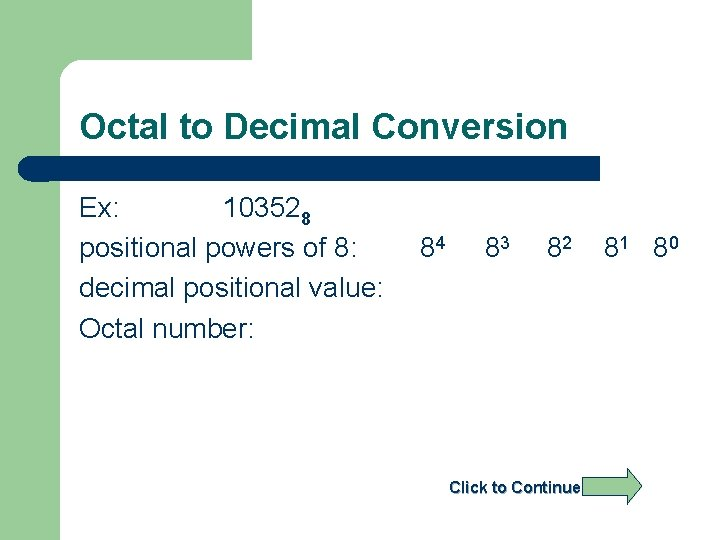Octal to Decimal Conversion Ex: 103528 positional powers of 8: decimal positional value: Octal number: 84 83 82 Click to Continue 8 1 80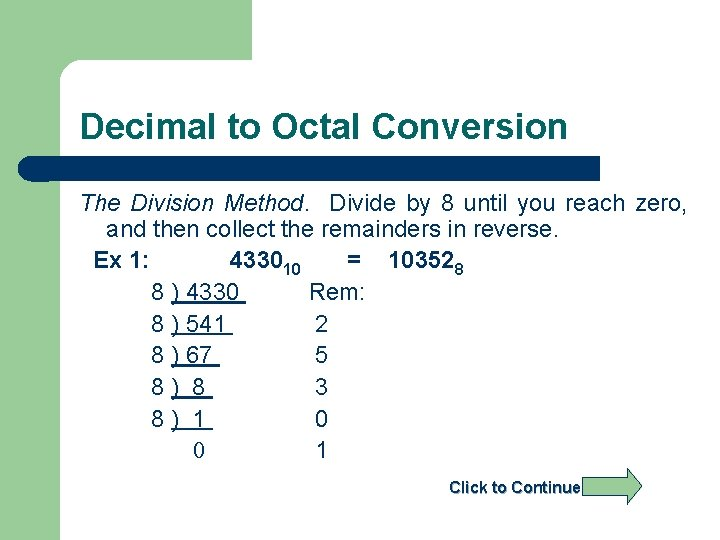Decimal to Octal Conversion The Division Method. Divide by 8 until you reach zero, and then collect the remainders in reverse. Ex 1: 433010 = 103528 8 ) 4330 Rem: 8 ) 541 2 8 ) 67 5 8) 8 3 8) 1 0 0 1 Click to Continue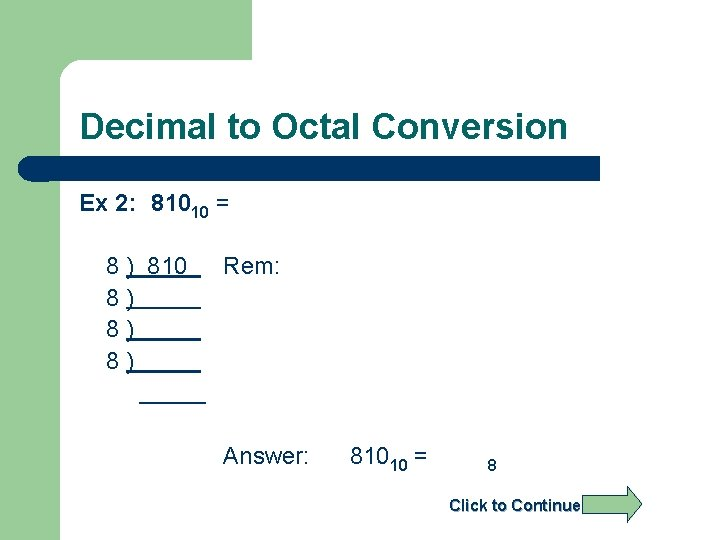Decimal to Octal Conversion Ex 2: 81010 = 8 ) 810 8) 8) 8) Rem: Answer: 81010 = 8 Click to Continue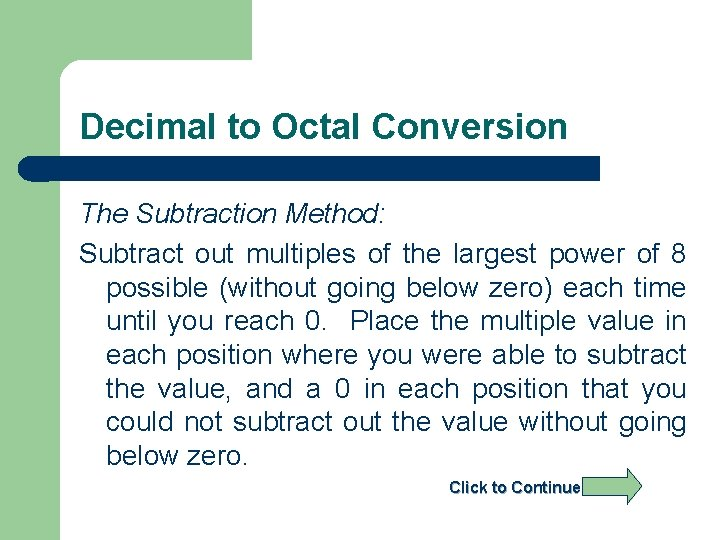Decimal to Octal Conversion The Subtraction Method: Subtract out multiples of the largest power of 8 possible (without going below zero) each time until you reach 0. Place the multiple value in each position where you were able to subtract the value, and a 0 in each position that you could not subtract out the value without going below zero. Click to ContinueDecimal to Octal Conversion Ex 1: 2018 - 1536 482 - 448 34 - 32 2 - 2 0 201810 84 | 83 82 81 80 4096 | 512 64 8 1 | 3 7 4 2 Answer: 201810 = 37428Decimal to Octal Conversion Ex 2: 76510 84 | 83 | | 82 Answer: 76510 = 81 80 8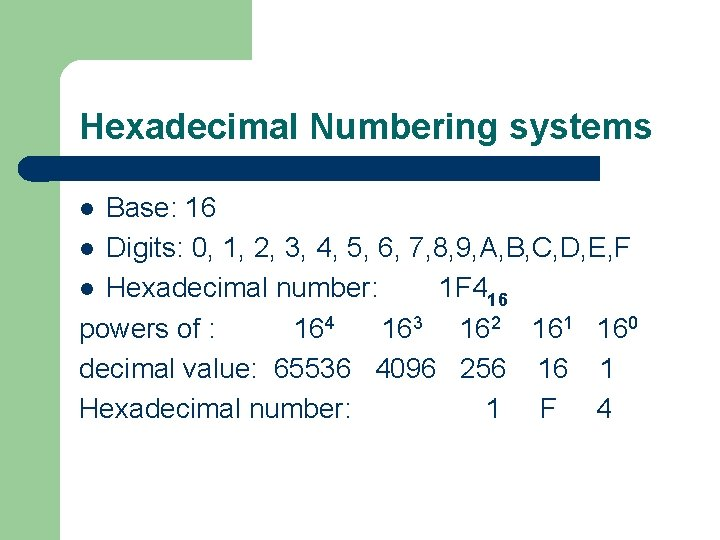Hexadecimal Numbering systems Base: 16 l Digits: 0, 1, 2, 3, 4, 5, 6, 7, 8, 9, A, B, C, D, E, F l Hexadecimal number: 1 F 416 powers of : 164 163 162 161 160 decimal value: 65536 4096 256 16 1 Hexadecimal number: 1 F 4 l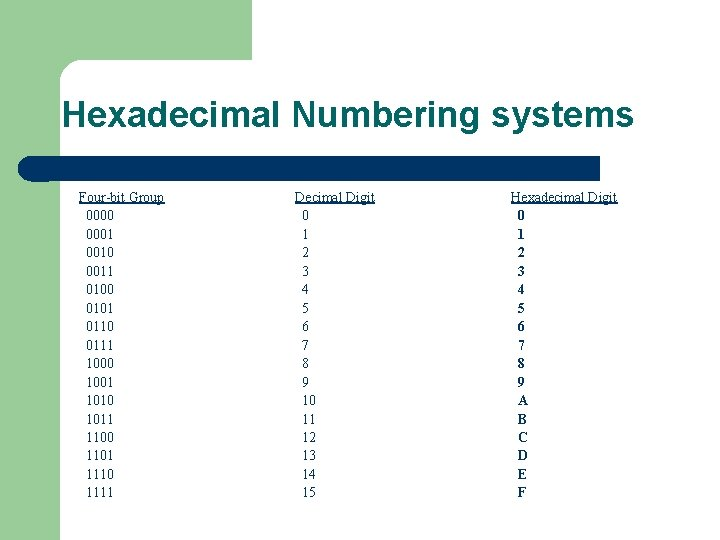Hexadecimal Numbering systems Four-bit Group 0000 0001 0010 0011 0100 0101 0110 0111 1000 1001 1010 1011 1100 1101 1110 1111 Decimal Digit 0 1 2 3 4 5 6 7 8 9 10 11 12 13 14 15 Hexadecimal Digit 0 1 2 3 4 5 6 7 8 9 A B C D E F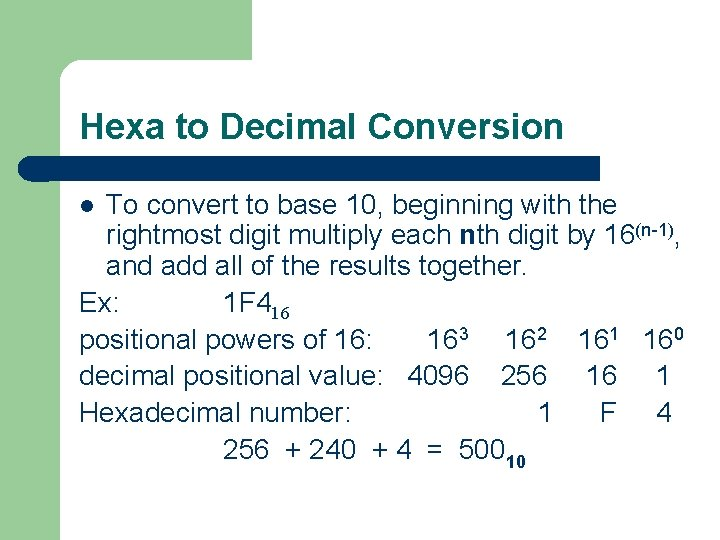Hexa to Decimal Conversion To convert to base 10, beginning with the rightmost digit multiply each nth digit by 16(n-1), and add all of the results together. Ex: 1 F 416 positional powers of 16: 163 162 161 160 decimal positional value: 4096 256 16 1 Hexadecimal number: 1 F 4 256 + 240 + 4 = 50010 lHexa to Decimal Conversion Ex: 7 E 16 positional powers of 16: decimal positional value: Hexa number: 163 162 161 160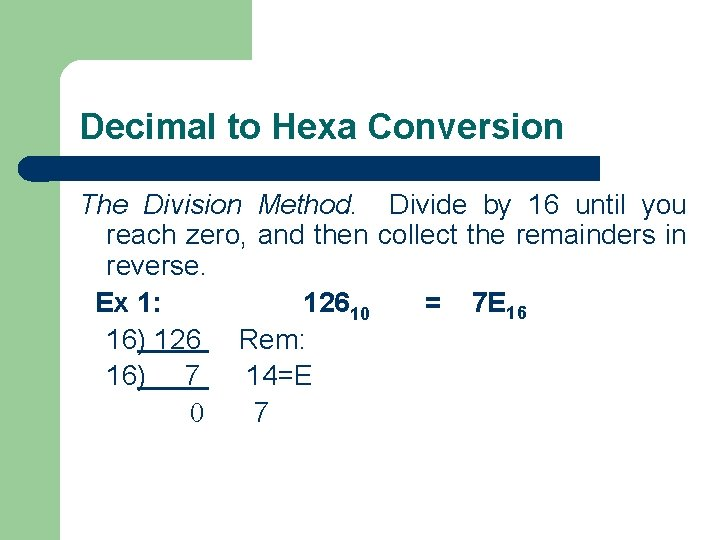Decimal to Hexa Conversion The Division Method. Divide by 16 until you reach zero, and then collect the remainders in reverse. Ex 1: 12610 = 7 E 16 16) 126 Rem: 16) 7 14=E 0 7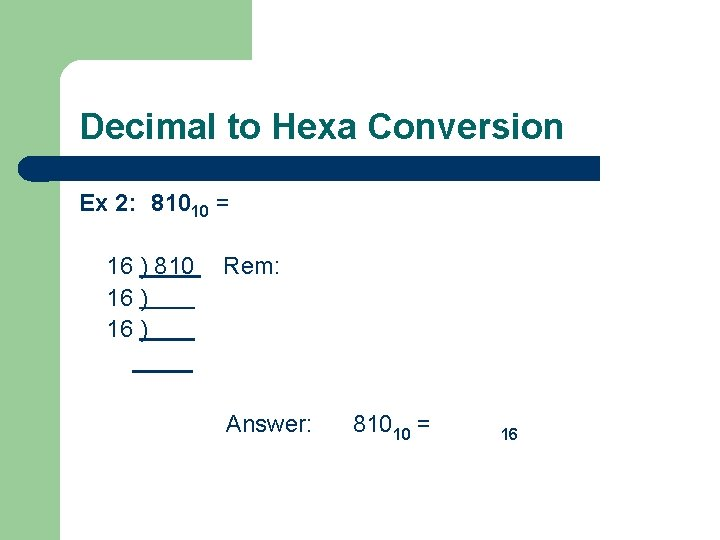Decimal to Hexa Conversion Ex 2: 81010 = 16 ) 810 16 ) Rem: Answer: 81010 = 16Decimal to Hexa Conversion The Subtraction Method: Subtract out multiples of the largest power of 16 possible (without going below zero) each time until you reach 0. Place the multiple value in each position where you were able to subtract the value, and a 0 in each position that you could not subtract out the value without going below zero.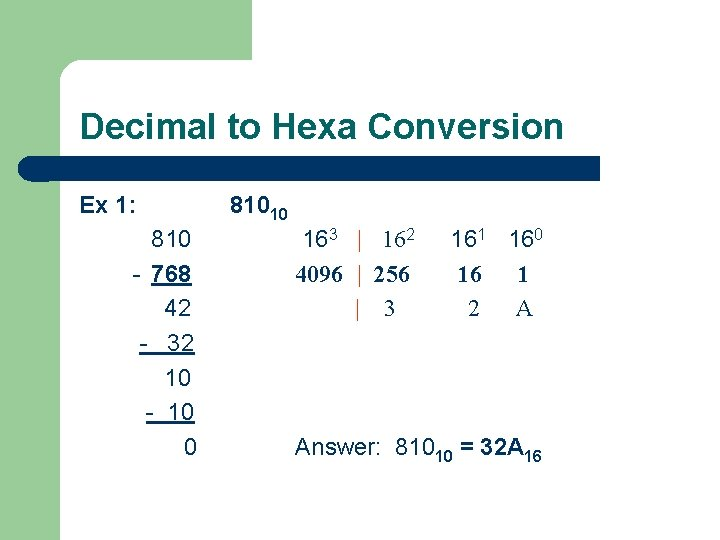Decimal to Hexa Conversion Ex 1: 810 - 768 42 - 32 10 - 10 0 81010 163 | 162 4096 | 256 | 3 161 160 16 1 2 A Answer: 81010 = 32 A 16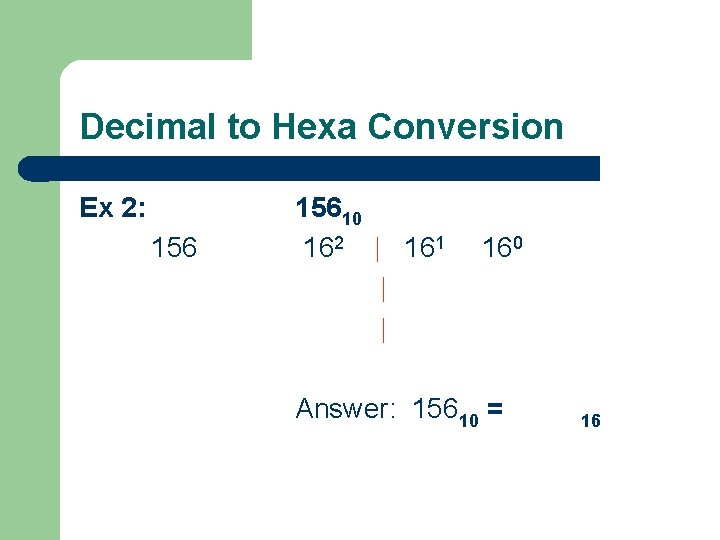Decimal to Hexa Conversion Ex 2: 15610 162 | 161 | | 160 Answer: 15610 = 16Binary to Octal Conversion Since the maximum value represented in 3 bit is equal to: 23 – 1 = 7 i. e. using 3 bits we can represent values from 0 – 7 which are the digits of the Octal numbering system. Thus, three binary digits can be converted to one octal digit.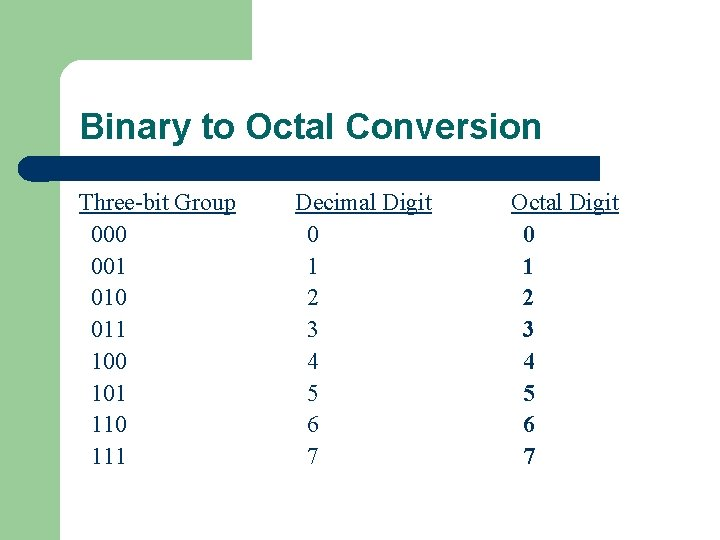Binary to Octal Conversion Three-bit Group 000 001 010 011 100 101 110 111 Decimal Digit 0 1 2 3 4 5 6 7 Octal Digit 0 1 2 3 4 5 6 7Octal to Binary Conversion Ex : Convert 7428 = 2 7 = 111 4 = 100 2 = 010 7428 = 111 100 0102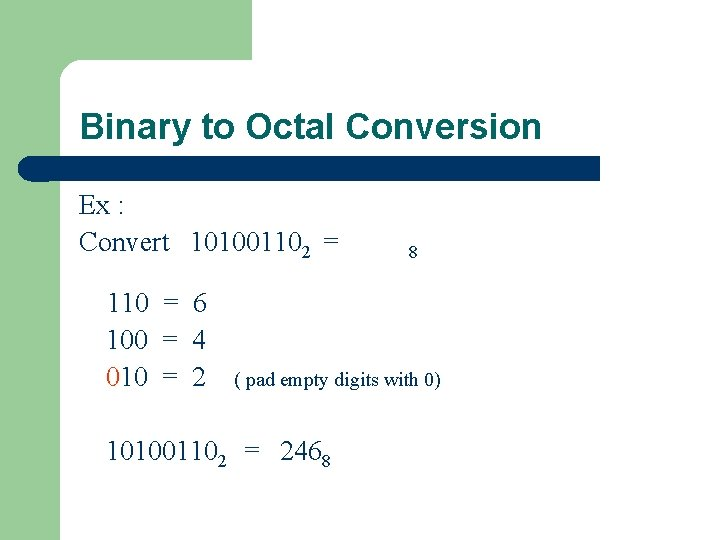Binary to Octal Conversion Ex : Convert 101001102 = 110 = 6 100 = 4 010 = 2 8 ( pad empty digits with 0) 101001102 = 2468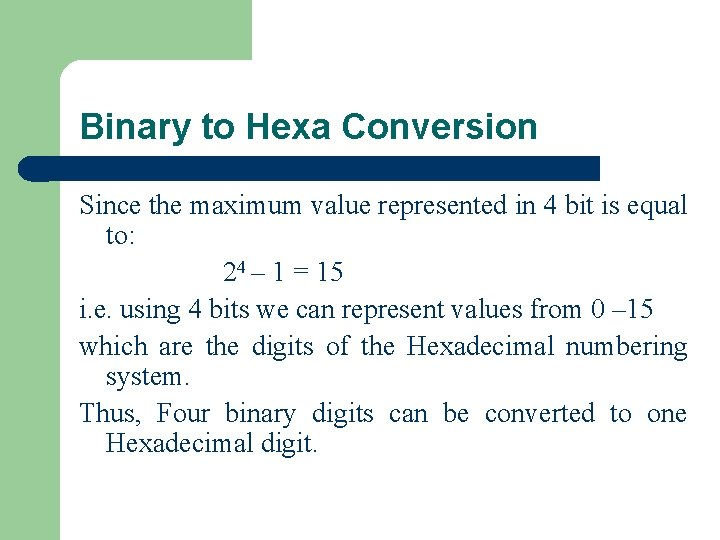Binary to Hexa Conversion Since the maximum value represented in 4 bit is equal to: 24 – 1 = 15 i. e. using 4 bits we can represent values from 0 – 15 which are the digits of the Hexadecimal numbering system. Thus, Four binary digits can be converted to one Hexadecimal digit.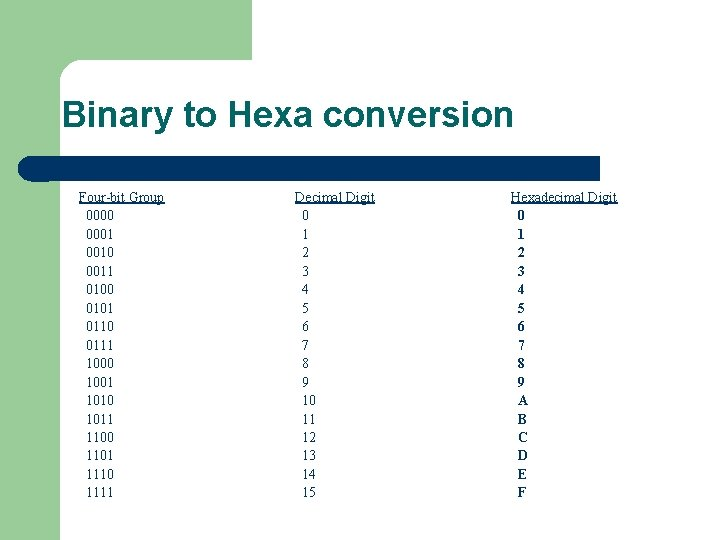Binary to Hexa conversion Four-bit Group 0000 0001 0010 0011 0100 0101 0110 0111 1000 1001 1010 1011 1100 1101 1110 1111 Decimal Digit 0 1 2 3 4 5 6 7 8 9 10 11 12 13 14 15 Hexadecimal Digit 0 1 2 3 4 5 6 7 8 9 A B C D E FHexa to Binary Conversion Ex : Convert 3 D 98 = 2 3 = 0011 D = 1101 9 = 1001 3 D 98 = 0011 1101 10012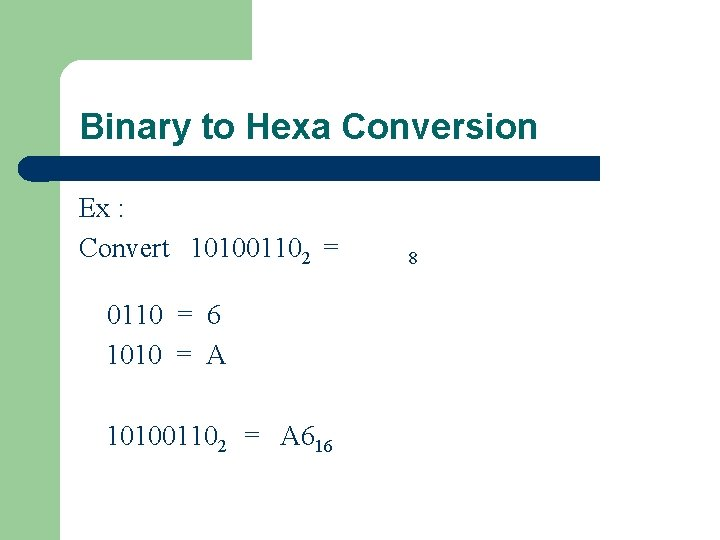Binary to Hexa Conversion Ex : Convert 101001102 = 0110 = 6 1010 = A 101001102 = A 616 8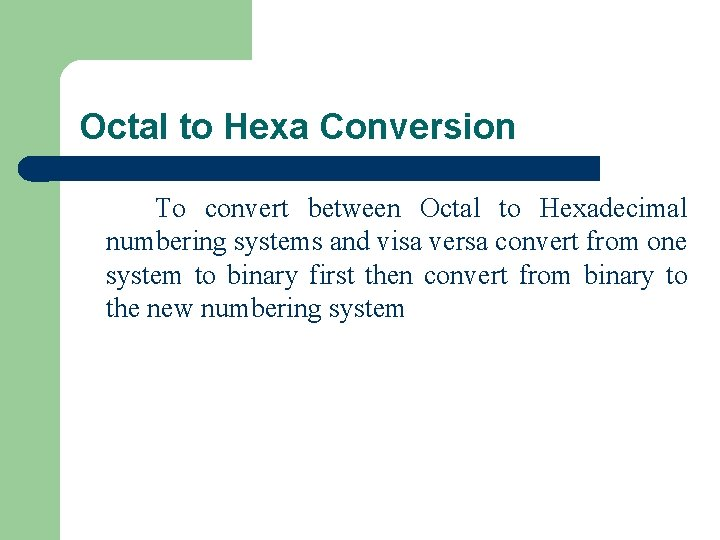Octal to Hexa Conversion To convert between Octal to Hexadecimal numbering systems and visa versa convert from one system to binary first then convert from binary to the new numbering system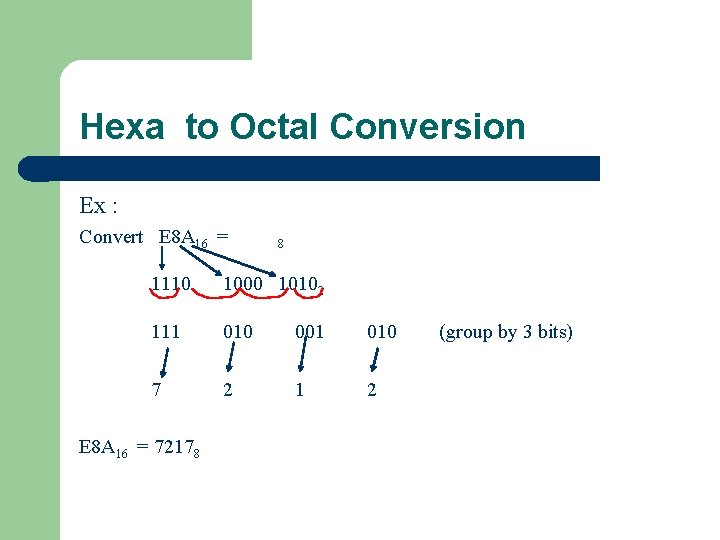Hexa to Octal Conversion Ex : Convert E 8 A 16 = 8 1110 1000 10102 111 010 001 010 7 2 1 2 E 8 A 16 = 72178 (group by 3 bits)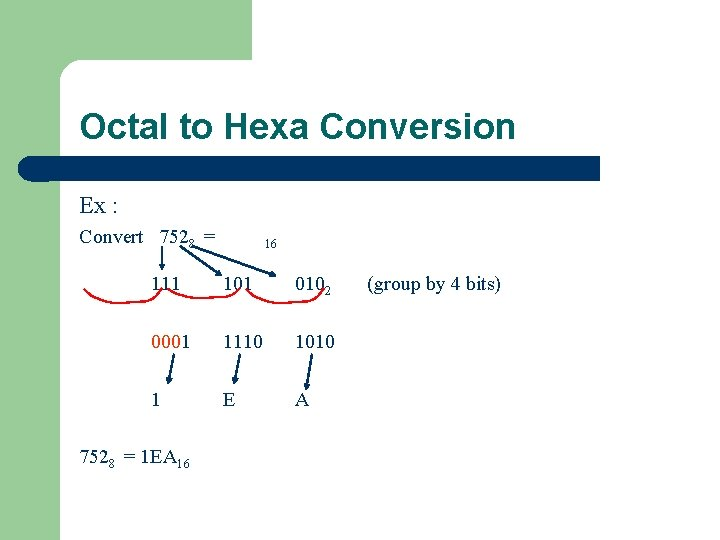Octal to Hexa Conversion Ex : Convert 7528 = 16 111 101 0102 0001 1110 1010 1 E A 7528 = 1 EA 16 (group by 4 bits)